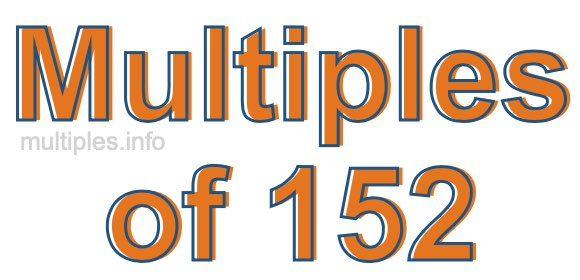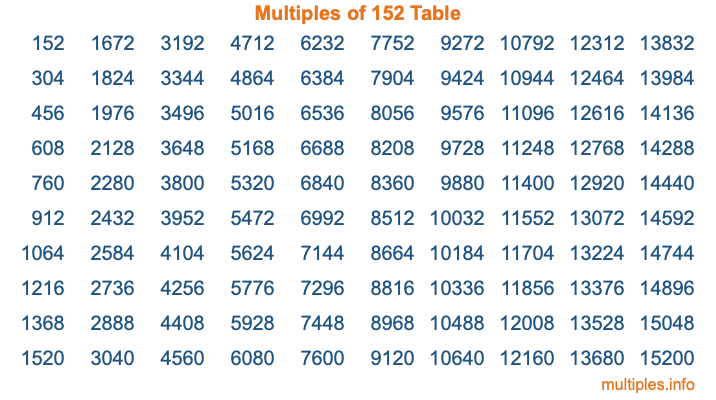Multiples of 152Welcome to the Multiples of 152 page. Here we will first teach you everything you will ever need to know about the multiples of 152, and then give you a study guide summary of everything we taught you to make sure you remember it all. Use this page to look up facts and learn information about the multiples of 152. This page will make you a multiples of one hundred fifty-two expert!

Definition of Multiples of 152
Multiples of 152 are all the numbers that when divided by 152 equal an integer. Each of the multiples of 152 are called a multiple. A multiple of 152 is created by multiplying 152 by an integer.

Therefore, to create a list of multiples of 152, you start with 1 multiplied by 152, then 2 multiplied by 152, then 3 multiplied by 152, and so on for as long as you want. Thus, the list of the first five multiples of 152 is 152, 304, 456, 608, and 760. To see a larger list of multiples of 152, see the printable image of Multiples of 152 further down on this page. We also have a category where you can choose any nth multiple of 152.

Multiples of 152 Checker
The Multiples of 152 Checker below checks to see if any number of your choice is a multiple of 152. In other words, it checks to see if there is any number (integer) that when multiplied by 152 will equal your number. To do that, we divide your number by 152. If the the quotient is an integer, then your number is a multiple of 152.

Is  a multiple of 152?

Least Common Multiple of 152 and ...
A Least Common Multiple (LCM) is the lowest multiple that two or more numbers have in common. This is also called the smallest common multiple or lowest common multiple and is useful to know when you are adding our subtracting fractions. Enter one or more numbers below (152 is already entered) to find the LCM.

Check out our LCM Calculator if you need more details about the Least Common Multiple or if you need the LCM for different numbers for adding and subtraction fractions.

nth Multiple of 152
As we stated above, 152 is the first multiple of 152, 304 is the second multiple of 152, 456 is the third multiple of 152, and so on. Enter a number below to find the nth multiple of 152.

th multiple of 152

Multiples of 152 vs Factors of 152
152 is a multiple of 152 and a factor of 152, but that is where the similarities end. All postive multiples of 152 are 152 or greater than 152. All positive factors of 152 are 152 or less than 152.

Below is the beginning list of multiples of 152 and the factors of 152 so you can compare:

Multiples of 152: 152, 304, 456, 608, 760, etc.

Factors of 152: 1, 2, 4, 8, 19, 38, 76, 152

As you can see, the multiples of 152 are all the numbers that you can divide by 152 to get a whole number. The factors of 152, on the other hand, are all the whole numbers that you can multiply by another whole number to get 152.

It's also interesting to note that if a number (x) is a factor of 152, then 152 will also be a multiple of that number (x).

Multiples of 152 vs Divisors of 152
The divisors of 152 are all the integers that 152 can be divided by evenly. Below is a list of the divisors of 152.

Divisors of 152: 1, 2, 4, 8, 19, 38, 76, 152

The interesting thing to note here is that if you take any multiple of 152 and divide it by a divisor of 152, you will see that the quotient is an integer.

Multiples of 152 Table
Below is an image of the first 100 multiples of 152 in a table. The table is in chronological order, column by column. The first column has the first ten multiples of 152, the second column has the next ten multiples of 152, and so on.The Multiples of 152 Table is also referred to as the 152 Times Table or Times Table of 152. You are welcome to print out our table for your studies.

Negative Multiples of 152
Although not often discussed or needed in math, it is worth mentioning that you can make a list of negative multiples of 152 by multiplying 152 by -1, then by -2, then by -3, and so on, to get the following list of negative multiples of 152:

-152, -304, -456, -608, -760, etc.

Multiples of 152 Summary
Below is a summary of important Multiples of 152 facts that we have discussed on this page. To retain the knowledge on this page, we recommend that you read through the summary and explain to yourself or a study partner why they hold true.

There are an infinite number of multiples of 152.

A multiple of 152 divided by 152 will equal a whole number.

152 divided by a factor of 152 equals a divisor of 152.

The nth multiple of 152 is n times 152.

The largest factor of 152 is equal to the first positive multiple of 152.

152 is a multiple of every factor of 152.

152 is a multiple of 152.

A multiple of 152 divided by a divisor of 152 equals an integer.

152 divided by a divisor of 152 equals a factor of 152.

Any integer times 152 will equal a multiple of 152.

Multiples of a Number
Here you can get the multiples of another number, all with the same attention to detail as we did for multiples of 152 on this page.

Multiples of
Multiples of 153
Did you find our page about multiples of one hundred fifty-two educational? Do you want more knowledge? Check out the multiples of the next number on our list!